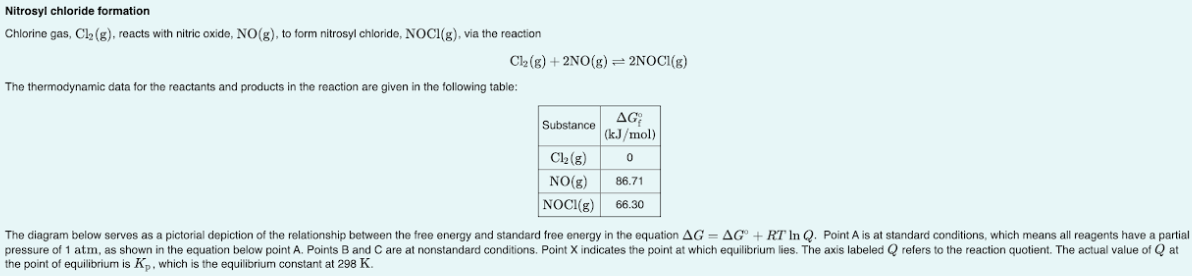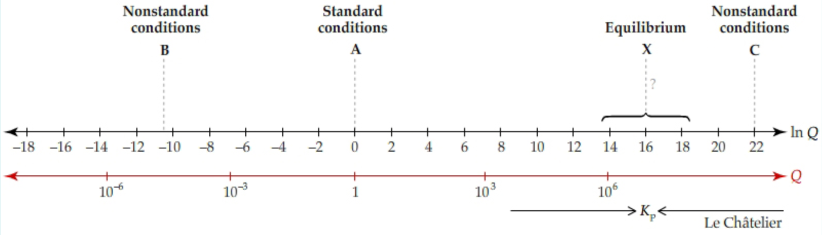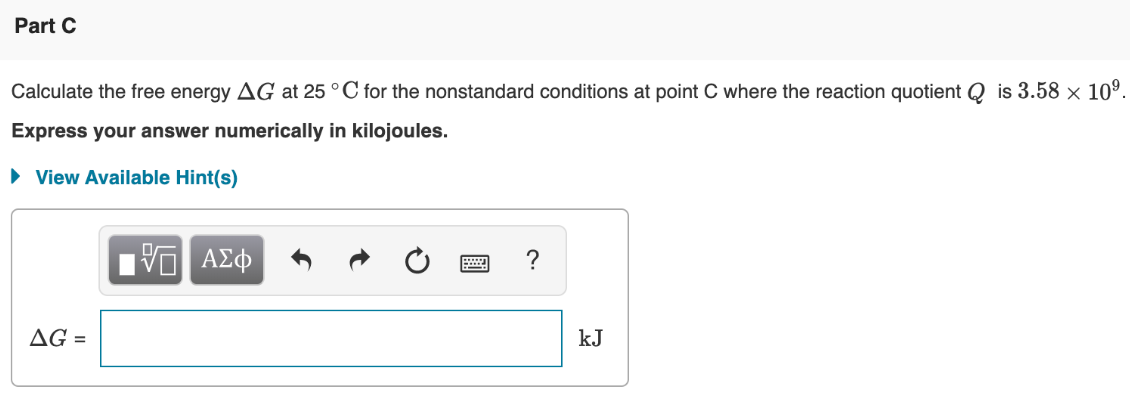# Chlorine gas, Cl2(g), reacts with nitric oxide, NO(g), to form nitrosyl chloride, NOCl(g), via the reaction Cl2(g) + 2NO(g) ⇌ 2NOCl(g) The thermodynamic data for the reactants and products in the reaction are given in the following table: The diagram below serves as a pictorial depiction of the relationship between the free energy and standard free energy in the equation ΔG = ΔG° + RT ln Q. Point A is at standard conditions, which means all reagents have a partial pressure of 1 atm, as shown in the equation below point A. Points B and C are at nonstandard conditions. Point X indicates the point at which equilibrium lies. The axis labeled Q refers to the reaction quotient. The actual value of Q at the point of equilibrium is Kp, which is the equilibrium constant at 298 K. Calculate the free energy ΔG at 25 °C for the nonstandard conditions at point C where the reaction quotient Q is 3.58 x 10^9. Express your answer numerically in kilojoules.